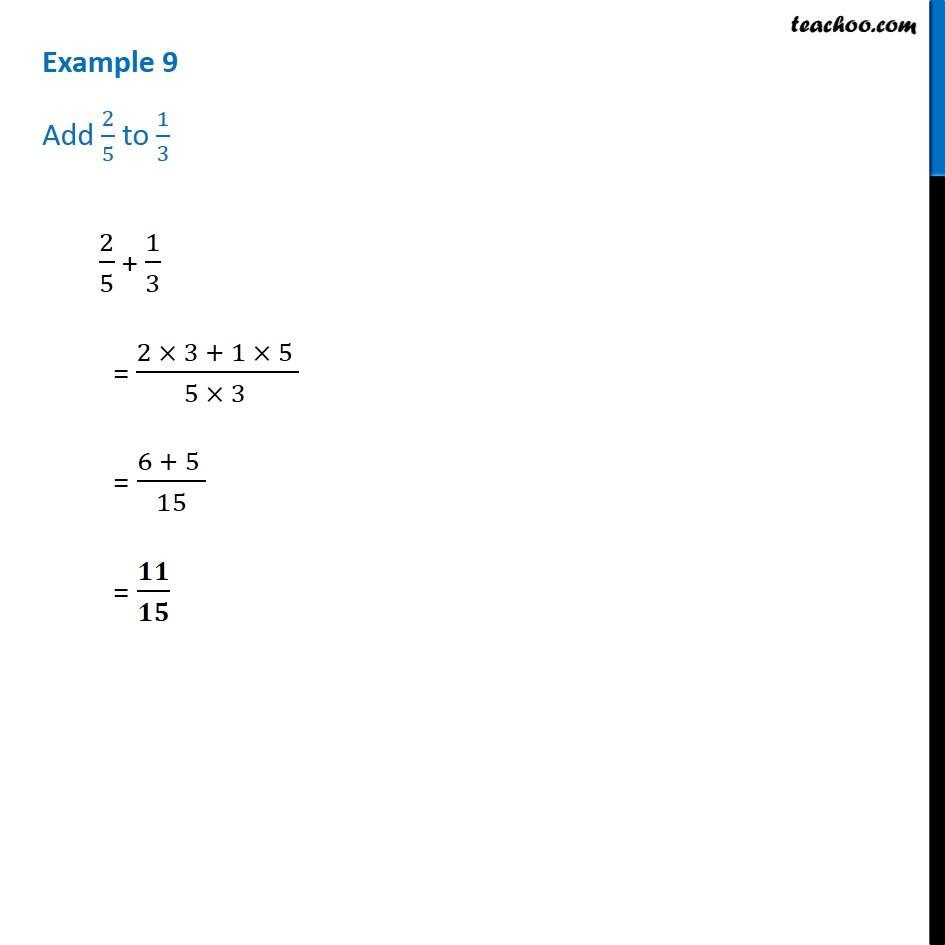1. Chapter 7 Class 6 Fractions
2. Concept wise
3. Addition and Subtraction of Unlike Fractions

Transcript

Example 9 - Chapter 7 Class 6 NCERT - Fractions Add 2/5 to 1/3 2/5 + 1/3 = (2 × 3 + 1 × 5)/(5 × 3) = (6 + 5)/15 = 11/15

Addition and Subtraction of Unlike Fractions